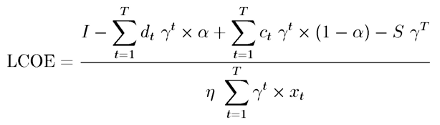# The Levelized Cost of Electricity

## Introduction

Cost of energy production remains perhaps the single most important factor in determining whether an energy technology can reach commercialization. To properly gauge the cost of a specific energy producing technology, we need to develop a standard by which we can compare any variety of technologies. The levelized cost of electricity (LCOE) serves as such a metric. All relevant parameters are factored into the calculation and the end result is a price per kilowatt-hour.

## Calculation

The LCOE is a long term cost concept which accounts for all the resources and physical assets required to yield a stream of electricity output. The LCOE represents a "break-even" value that a power provider would need to charge in order to justify an investment in a particular energy project .

From a high level we can express the LCOE as the following:

 LCOE = Total Life Cycle CostTotal Lifetime Energy Production = I - D + C - SE

I represents the initial investment, D represents a depreciation tax shield, C represents annual cost, S is the salvage value of any physical assets at the end of life-cycle and E is the total energy production. We can disaggregate the above equation to yield the following full expression for the LCOE:The factor γ represents the time-value of money [γ ≡ 1/(1+r)], α is the corporate tax rate, η is the energy production, and T is the life-cycle of the project. We choose dt, ct and xt to represent the depreciation schedule in year t, the operating cost in year t, and the system degradation in year t, respectively .

## Example: Natural Gas (NGCC) Plant

### System Estimates 

• Useful life of NGCC plant is T = 30 years

• Productive capacity remains undiminished (xt = 1) for entire 30 years

• System price is \$360 million for a 550 MW plant

• Unit operating cost is w = 0.048 \$/kWh

• IRS allows 150% declining balance depreciation schedule

• Corporate tax rate is 40%

• Firms impute a weighted average cost of capital (WACC) of r = 0.08

### LCOE for NGCC Plant

Using the above parameters we calculate that for a new natural gas NGCC plant, the Levelized Cost of Electricity is 0.058 \$/kWh.

© Alok Vasudev. The author grants permission to copy, distribute and display this work in unaltered form, with attribution to the author, for noncommercial purposes only. All other rights, including commercial rights, are reserved to the author.

## References

 Ö. Islegen and S. Reichelstein, "Carbon Capture by Fossil Fuel Power Plants: An Economic Analysis," Management Sci. 57, 21 (2011).

 M. Campbell, "The Drivers of the Levelized Cost of Electricity for Utility-Scale Photovoltaics," SunPower Corporation, February 2008.

 "Cost and Performance Baseline for fossil Energy Plants - Vol. 1: Bituminous Coal and natural Gas to Electricity," U.S. National Energy Technology laboratory," DOE/NETL-2010/1397, November 2010.